Download Presentation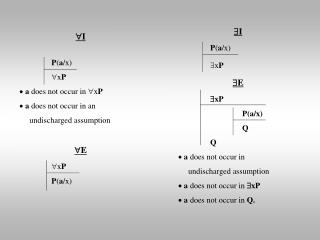 I P ( a /x)  x P a does not occur in  x P a does not occur in an

#  I P ( a /x)  x P a does not occur in  x P a does not occur in an - PowerPoint PPT Presentation

 I P ( a /x)  x P  E  xP P(a/x) Q Q a does not occur in undischarged assumption a does not occur in  xP a does not occur in Q.  I P ( a /x)  x P a does not occur in  x P a does not occur in an undischarged assumption  E  x P P ( a /x).  II am the owner, or an agent authorized to act on behalf of the owner, of the copyrighted work described.
Download Presentation##  I P ( a /x)  x P a does not occur in  x P a does not occur in an

An Image/Link below is provided (as is) to download presentation

Download Policy: Content on the Website is provided to you AS IS for your information and personal use and may not be sold / licensed / shared on other websites without getting consent from its author.While downloading, if for some reason you are not able to download a presentation, the publisher may have deleted the file from their server.

- - - - - - - - - - - - - - - - - - - - - - - - - - E N D - - - - - - - - - - - - - - - - - - - - - - - - - -
Presentation Transcript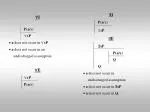I

• P(a/x)
• xP
• E
• xP
• P(a/x)
• Q
• Q
• a does not occur in
• undischarged assumption
• a does not occur inxP
• a does not occur in Q.
• I
• P(a/x)
• xP
• a does not occur in xP
• a does not occur in an
• undischarged assumption
• E
• xP
• P(a/x)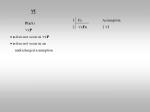I

• P(a/x)
• xP
• a does not occur in xP
• a does not occur in an
• undischarged assumption
• Fa Assumption
• xFx 2 I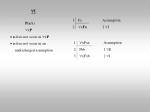I

• P(a/x)
• xP
• a does not occur in xP
• a does not occur in an
• undischarged assumption
• Fa Assumption
• xFx 2 I
• xFxx Assumption
• Fbb 1 E
• xFxb 2 I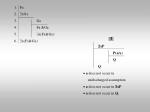Fa

• xGx
• Ga
• Fa &Ga
• x(Fx&Gx)
• x(Fx&Gx)
• E
• xP
• P(a/x)
• Q
• Q
• a does not occur in
• undischarged assumption
• a does not occur inxP
• a does not occur in Q.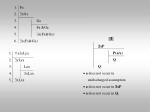Fa

• xGx
• Ga
• Fa &Ga
• x(Fx&Gx)
• x(Fx&Gx)
• E
• xP
• P(a/x)
• Q
• Q
• a does not occur in
• undischarged assumption
• a does not occur inxP
• a does not occur in Q.
• xyLyx
• yLya
• Laa
• xLxx
• xLxx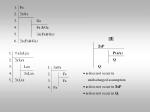Fa

• xGx
• Ga
• Fa &Ga
• x(Fx&Gx)
• x(Fx&Gx)
• E
• xP
• P(a/x)
• Q
• Q
• a does not occur in
• undischarged assumption
• a does not occur inxP
• a does not occur in Q.
• xyLyx
• yLya
• Laa
• xLxx
• xLxx
• xFx
• Fa
• Fa
• Fa